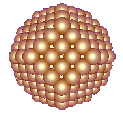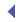Figurate Numbers Figurate numbers take the shape of regular two-dimensional polygons and three-dimensional polyhedra. Each type of figurate number has a formula associated with it. For instance, n² generates square numbers. The first few square numbers are 1, 4, 9, 16, 25, 36, 49, 64, 81, 100. . . . The sixth square: 6² = 36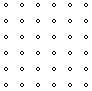(n² +n)/2 generates triangle numbers. The fifth triangle: (5² +5)/2 = 15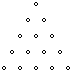(6n² –6n +1) generates star (hexagram) numbers. The third star, (6 × 3² –6 × 3 +1) = 37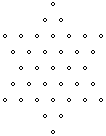There are also three-dimensional figurate numbers, such as truncated octahedrons, (16n³ –33n² + 24n –6). The fourth truncated octahedron: (16 × 4³ –33 × 4² + 24 × 4 –6) = 586